/

### Enterprise Risk Management Formula Book Appendix A.5: Probability Distributions: Multivariate probability distributions

Multivariate normal (i.e. Gaussian) distribution

The multivariate probability distribution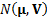where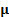is a vector ofelements and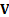is an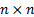non-negative definite matrix has the following joint density function (where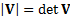is the determinant of V)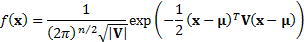The means of the individual marginal distributions are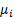where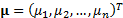and the covariance between the’th and the’th marginal distributions are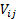where theare the elements of. Its moment generating function is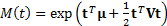and its characteristic function is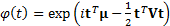. The multivariate normal distribution has as its copula the Gaussian copula.

A bivariate random variable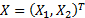follows a standard bivariate normal distribution if it has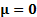and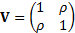. More generally, a multivariate normal distribution is a standard multivariate normal distribution ifand a covariance matrix which is also a correlation matrix, i.e. where the variance of each individual marginal distribution is 1.

For numerical values of the cumulative distribution function of the standard bivariate normal distribution see here.